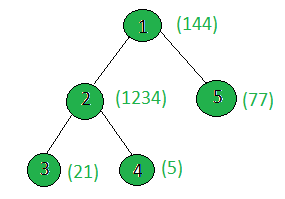# Count the nodes in the given tree whose sum of digits of weight is odd

Given a tree, and the weights of all the nodes, the task is to count the number of nodes whose sum of digits of weights is odd.

Examples:

Input:Output: 3
Node 1: digitSum(144) = 1 + 4 + 4 = 9
Node 2: digitSum(1234) = 1 + 2 + 3 + 4 = 10
Node 3: digitSum(21) = 2 + 1 = 3
Node 4: digitSum(5) = 5
Node 5: digitSum(77) = 7 + 7 = 14
Only the sum of digits of the weights of nodes 1, 3 and 4 are odd.

## Recommended: Please try your approach on {IDE} first, before moving on to the solution.

Approach: Perform dfs on the tree and for every node, check if the sum of the digits of its weight is odd. If yes then increment the count.

Below is the implementation of the above approach:

## C++

 `// C++ implementation of the approach ` `#include ` `using` `namespace` `std; ` ` `  `int` `ans = 0; ` ` `  `vector<``int``> graph; ` `vector<``int``> weight(100); ` ` `  `// Function to return the ` `// sum of the digits of n ` `int` `digitSum(``int` `n) ` `{ ` `    ``int` `sum = 0; ` `    ``while` `(n) { ` `        ``sum += n % 10; ` `        ``n = n / 10; ` `    ``} ` `    ``return` `sum; ` `} ` ` `  `// Function to perform dfs ` `void` `dfs(``int` `node, ``int` `parent) ` `{ ` `    ``// If sum of the digits of current node's ` `    ``// weight is odd then increment ans ` `    ``int` `sum = digitSum(weight[node]); ` `    ``if` `(sum % 2 == 1) ` `        ``ans += 1; ` ` `  `    ``for` `(``int` `to : graph[node]) { ` `        ``if` `(to == parent) ` `            ``continue``; ` `        ``dfs(to, node); ` `    ``} ` `} ` ` `  `// Driver code ` `int` `main() ` `{ ` ` `  `    ``// Weights of the node ` `    ``weight = 144; ` `    ``weight = 1234; ` `    ``weight = 21; ` `    ``weight = 5; ` `    ``weight = 77; ` ` `  `    ``// Edges of the tree ` `    ``graph.push_back(2); ` `    ``graph.push_back(3); ` `    ``graph.push_back(4); ` `    ``graph.push_back(5); ` ` `  `    ``dfs(1, 1); ` ` `  `    ``cout << ans; ` ` `  `    ``return` `0; ` `} `

## Java

 `// Java implementation of the approach ` `import` `java.util.*; ` ` `  `class` `GFG  ` `{ ` `    ``static` `int` `ans = ``0``; ` ` `  `    ``static` `Vector[] graph = ``new` `Vector[``100``]; ` `    ``static` `Integer[] weight = ``new` `Integer[``100``]; ` ` `  `    ``// Function to return the ` `    ``// sum of the digits of n ` `    ``static` `int` `digitSum(``int` `n)  ` `    ``{ ` `        ``int` `sum = ``0``; ` `        ``while` `(n > ``0``)  ` `        ``{ ` `            ``sum += n % ``10``; ` `            ``n = n / ``10``; ` `        ``} ` `        ``return` `sum; ` `    ``} ` ` `  `    ``// Function to perform dfs ` `    ``static` `void` `dfs(``int` `node, ``int` `parent) ` `    ``{ ` `         `  `        ``// If sum of the digits of current node's ` `        ``// weight is odd then increment ans ` `        ``int` `sum = digitSum(weight[node]); ` `        ``if` `(sum % ``2` `== ``1``) ` `            ``ans += ``1``; ` ` `  `        ``for` `(``int` `to : graph[node]) ` `        ``{ ` `            ``if` `(to == parent) ` `                ``continue``; ` `            ``dfs(to, node); ` `        ``} ` `    ``} ` ` `  `    ``// Driver code ` `    ``public` `static` `void` `main(String[] args) ` `    ``{ ` `        ``for` `(``int` `i = ``0``; i < ``100``; i++) ` `            ``graph[i] = ``new` `Vector(); ` `         `  `        ``// Weights of the node ` `        ``weight[``1``] = ``144``; ` `        ``weight[``2``] = ``1234``; ` `        ``weight[``3``] = ``21``; ` `        ``weight[``4``] = ``5``; ` `        ``weight[``5``] = ``77``; ` ` `  `        ``// Edges of the tree ` `        ``graph[``1``].add(``2``); ` `        ``graph[``2``].add(``3``); ` `        ``graph[``2``].add(``4``); ` `        ``graph[``1``].add(``5``); ` ` `  `        ``dfs(``1``, ``1``); ` ` `  `        ``System.out.print(ans); ` `    ``} ` `} ` ` `  `// This code is contributed by Rajput-Ji `

## C#

 `// C# implementation of the approach ` `using` `System; ` `using` `System.Collections.Generic; ` ` `  `class` `GFG  ` `{ ` `    ``static` `int` `ans = 0; ` ` `  `    ``static` `List<``int``>[] graph = ``new` `List<``int``>; ` `    ``static` `int``[] weight = ``new` `int``; ` ` `  `    ``// Function to return the ` `    ``// sum of the digits of n ` `    ``static` `int` `digitSum(``int` `n)  ` `    ``{ ` `        ``int` `sum = 0; ` `        ``while` `(n > 0)  ` `        ``{ ` `            ``sum += n % 10; ` `            ``n = n / 10; ` `        ``} ` `        ``return` `sum; ` `    ``} ` ` `  `    ``// Function to perform dfs ` `    ``static` `void` `dfs(``int` `node, ``int` `parent) ` `    ``{ ` `         `  `        ``// If sum of the digits of current node's ` `        ``// weight is odd then increment ans ` `        ``int` `sum = digitSum(weight[node]); ` `        ``if` `(sum % 2 == 1) ` `            ``ans += 1; ` ` `  `        ``foreach` `(``int` `to ``in` `graph[node]) ` `        ``{ ` `            ``if` `(to == parent) ` `                ``continue``; ` `            ``dfs(to, node); ` `        ``} ` `    ``} ` ` `  `    ``// Driver code ` `    ``public` `static` `void` `Main(String[] args) ` `    ``{ ` `        ``for` `(``int` `i = 0; i < 100; i++) ` `            ``graph[i] = ``new` `List<``int``>(); ` `         `  `        ``// Weights of the node ` `        ``weight = 144; ` `        ``weight = 1234; ` `        ``weight = 21; ` `        ``weight = 5; ` `        ``weight = 77; ` ` `  `        ``// Edges of the tree ` `        ``graph.Add(2); ` `        ``graph.Add(3); ` `        ``graph.Add(4); ` `        ``graph.Add(5); ` ` `  `        ``dfs(1, 1); ` ` `  `        ``Console.Write(ans); ` `    ``} ` `} ` ` `  `// This code is contributed by PrinciRaj1992 `

Output:

```3
```

My Personal Notes arrow_drop_upIf you like GeeksforGeeks and would like to contribute, you can also write an article using contribute.geeksforgeeks.org or mail your article to contribute@geeksforgeeks.org. See your article appearing on the GeeksforGeeks main page and help other Geeks.

Please Improve this article if you find anything incorrect by clicking on the "Improve Article" button below.

Improved By : Rajput-Ji, princiraj1992

Article Tags :
Practice Tags :

Be the First to upvote.

Please write to us at contribute@geeksforgeeks.org to report any issue with the above content.